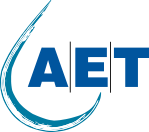# Oxygen transfer rate in Wastewater treatment – calculation example

Before we can calculate the oxygen transfer rate, we have to determine our oxygen demand. Depending on the wastewater treatment target different factors must be considered to calculate the actual oxygen requirement (AOR).

1. Design flows and loads: Water influents are not consistent and vary between daily minimum, average and peak flows. Design guidelines recommend dividing the average day of the peak month by 24 hours to calculate the needed aeration amount.
2. BOD load: The amount of oxygen needed for microorganisms to decompose organic material is about 0.9 to 1.3 lb O2 per lb BOD.
3. Total Nitrogen reduction: Nitrifying bacteria oxidizes ammonium (NH4+) first to Nitrite (No2-) and then to Nitrate (No3-). For this process the bacteria need about 4.6lb of O2 per pound of ammonium.

As an example, let’s assume we have an average day, peak month flow of 100,000GPD, 150ppm BOD and 40ppm ammonium in the influent of a wastewater treatment plant. Then the daily BOD loading is about 125lbs per day and the daily ammonium loading is about 30lbs per day. Therefore, the AOR per day is 125lbs * 1.1 + 30lbs * 4.6 = 275.5 lb oxygen.

Finally, the AOR must be corrected for environmental conditions such as temperature, pressure, O2 saturation and others. For wastewater conditions the correcting factor is usually between 0.3 and 0.5 depending on the aeration equipment. The corrected AOR value is known as the standard oxygen requirement (SOR). In our example we use a factor of 0.4 which results in an SOR of 688lbs O2 per day.

Now we know how much oxygen per day must be present in the water so that the biological processes can take place. However, we still have to transfer gaseous oxygen into the water to get to the calculated daily oxygen requirement. To dissolve gaseous oxygen into wastewater different products are developed by equipment manufacturers. Depending on the product technology the oxygen transfer rates differ between 0.5 and 2.5% per ft water depth.

The oxygen transfer rate (OTR) depends again on different factors such as the temperature, pressure, oxygen saturation and most important the alpha-factor which describes the differences of clean water to wastewater.

For our example lets assume we have 15ft water depth which equals an OTR efficiency of 1.5% * 15 = 22.5%. Therefore, the aeration equipment has to provide a total oxygen amount of 3,057lb per day.

Last, the required amount of oxygen has to be converted into the total required air amount as the aeration equipment does not provide pure oxygen. Air consists to 21% of oxygen therefore dividing our oxygen number of 3,057lb by 0.21 brings the total required air amount per day of 14,557lbs or 194,000ft3.